{{ message }}

# bmsleight / lasercut

Module for openscad, allowing 3d models to be created from 2d lasercut parts

Switch branches/tags
Nothing to show

## Files

Failed to load latest commit information.
Type
Name
Commit time
Feb 28, 2021
Aug 26, 2015
Feb 28, 2021
Oct 14, 2015
Aug 26, 2015
Aug 26, 2015
Feb 28, 2021

Module for openscad, allowing 3d models to be created from 2d lasercut parts, with a flat file automatically generated.

Updated, not just lasercut can now pass the parameter milling_bit for CNC machines, for the bit to cut in to the corners.

## Basic usage

To prepare a simple rectangle use lasercutoutSquare()

``````include <lasercut.scad>;

thickness = 3.1;
x = 50;
y = 100;
lasercutoutSquare(thickness=thickness, x=x, y=y);
``````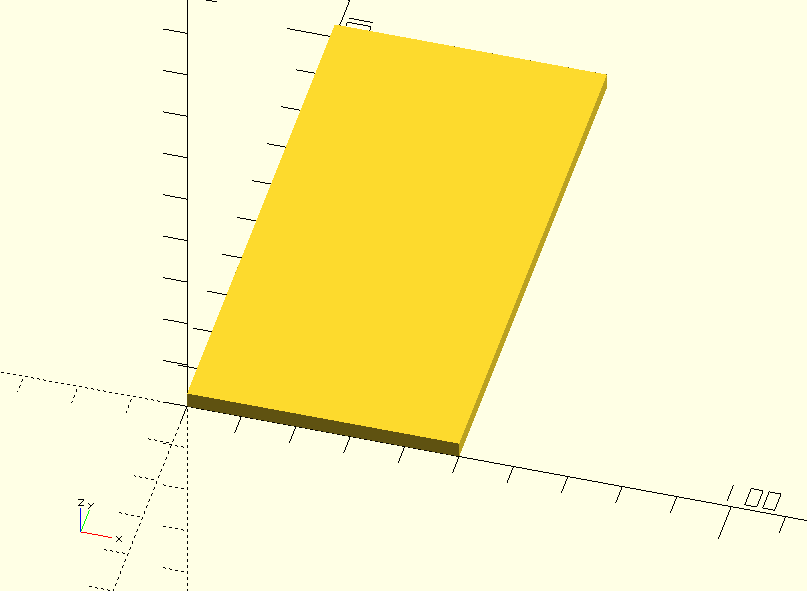More complex path using the lasercutout()

``````include <lasercut.scad>;

thickness = 3.1;
x = 50;
y = 100;
points = [[0,0], [x,0], [x,y], [x/2,y], [x/2,y/2], [0,y/2], [0,0]];
lasercutout(thickness=thickness, points = points);
``````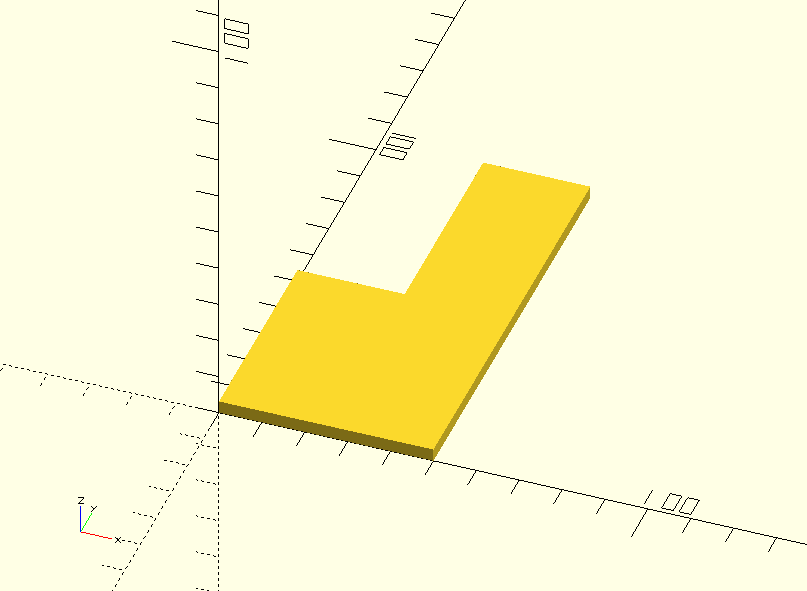## Simple Tab

``````include <lasercut.scad>;

thickness = 3.1;
x = 50;
y = 100;
lasercutoutSquare(thickness=thickness, x=x, y=y,
simple_tabs=[
[UP, x/2, y],
[DOWN, x/2, 0]
]
);

translate([0,y+20,-thickness]) rotate([90,0,0])
lasercutoutSquare(thickness=thickness, x=x, y=y,
simple_tab_holes=[
[MID, x/2-thickness/2, thickness]
]
);
``````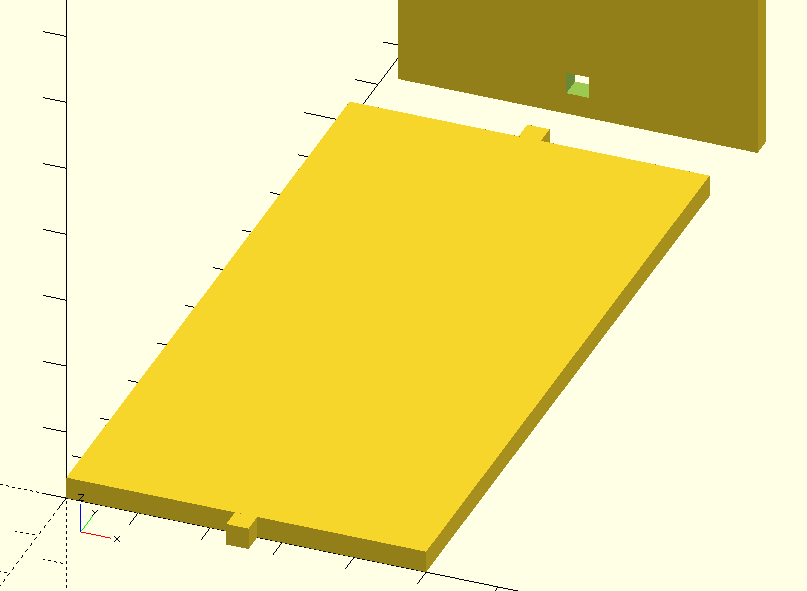## Captive Nuts

Great for holding laser-cut sheets together.

``````include <lasercut.scad>;

thickness = 3.1;
nut_flat_width = 9.3;
x = 50;
y = 100;
lasercutoutSquare(thickness=thickness, x=x, y=y,
simple_tabs=[
[UP, x/2, y],
[DOWN, x/2, 0]
],
captive_nuts=[
[LEFT, 0, y/2, nut_flat_width],
[RIGHT, x, y/2, nut_flat_width],

]
);

translate([0,y+20,-thickness]) rotate([90,0,0])
lasercutoutSquare(thickness=thickness, x=x, y=y,
simple_tab_holes=[
[MID, x/2-thickness/2, thickness]
]
);

translate([-20,0,0]) rotate([90,0,90])
lasercutoutSquare(thickness=thickness, x=y, y=x,
captive_nut_holes=[
[DOWN, y/2, 0]
]
);
``````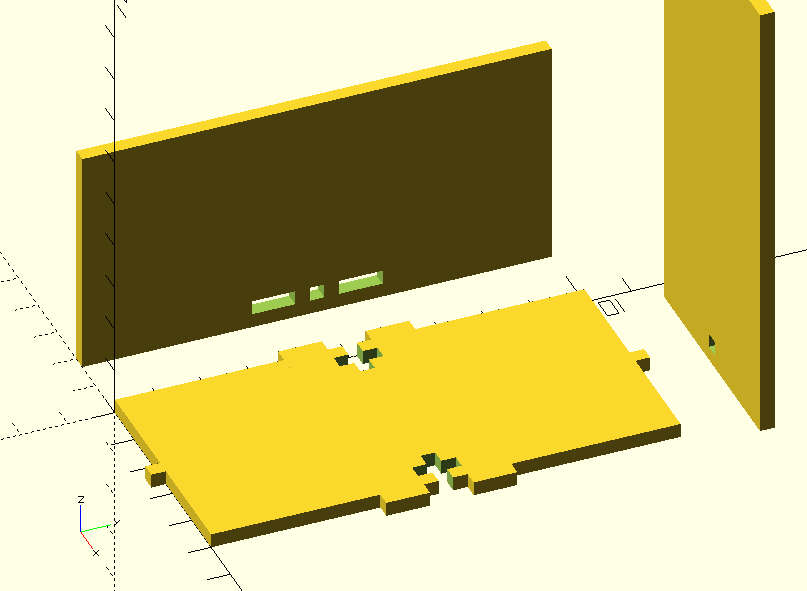## Box

Easy to make a box with four, five or six side. Uses finger joints with the correct alignment to give a flat edge regardless of number of sides.

``````include <lasercut.scad>;

thickness = 3.1;
x = 50;
y = 50;
z = 75;

for (sides =[4:6])
{
color("Gold",0.75) translate([100*(sides-4),0,0])
lasercutoutBox(thickness = thickness, x=x, y=y, z=z,
sides=sides, num_fingers=4);
}

``````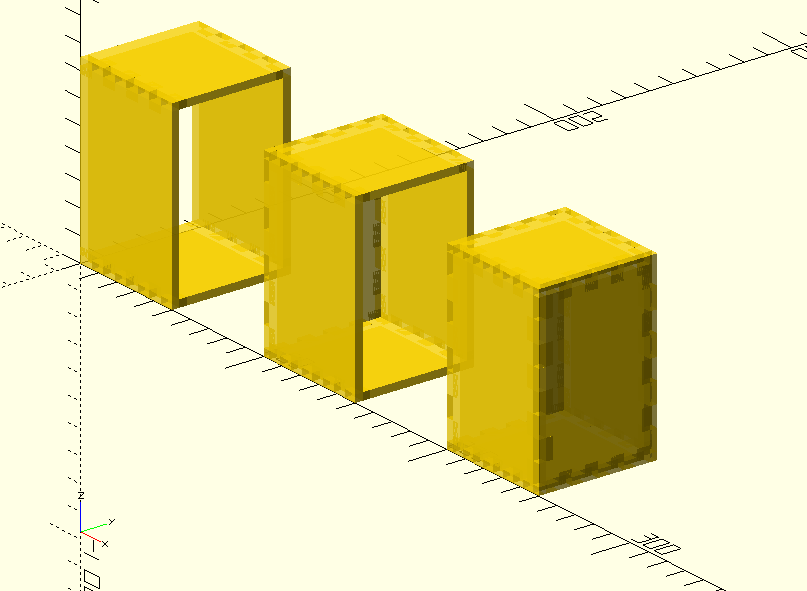## Box for Vinyl Cutters

Easy to make a box with four, five or six side. Uses finger joints with the correct alignment to give a flat edge regardless of number of sides.

``````include <lasercut.scad>;

lasercutoutVinylBox(x=60, y=50, z=80, thickness=0.2);
``````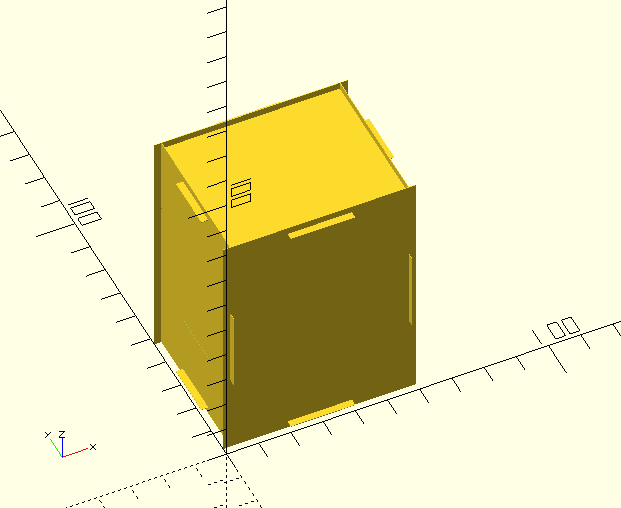More detail on Vinyl Boxes, including infil

## Finger Joints

Simple finger joints, (as automatically used in the box above). Parameters are direction, startup tap, even number) so for example [UP, 1, 4] - UP direction, starting with a tab not a gap, four figners.

As suggested in the OpenSCAD forum, bumpy_finger_joints, when printed in wood the dimple will crush a little and ensure a press fit.

``````include <../lasercut.scad>;

\$fn=60;
thickness = 3.1;
x = 50;
y = 100;
lasercutoutSquare(thickness=thickness, x=x, y=y,
bumpy_finger_joints=[
[UP, 1, 4],
[DOWN, 1, 4]
]
);

translate([0,y+20,thickness]) rotate([90,0,0])
lasercutoutSquare(thickness=thickness, x=x, y=y,
finger_joints=[
[UP, 1, 4],
[DOWN, 1, 4]
]
);

``````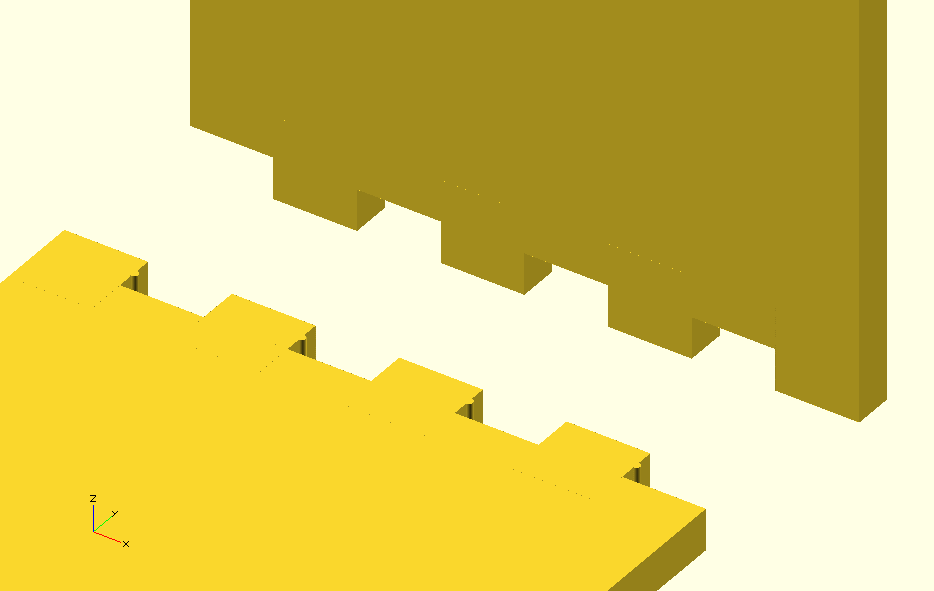## Finger Joints Milled

Simple finger joints, (as automatically used in the box above), but now using the parameter milling_bit for the bit to cut in to the corners.

``````include <../lasercut.scad>;

\$fn=60;
thickness = 3.1;
x = 130;
y = 100;
lasercutoutSquare(thickness=thickness, x=x, y=y,
finger_joints=[
[UP, 0, 2],
[DOWN, 1, 2]
],
milling_bit=3.125
);

translate([0,y+20,thickness]) lasercutoutBox(thickness = thickness, x=x, y=y, z=x/2, sides=5,
num_fingers=2, milling_bit=3.125);
``````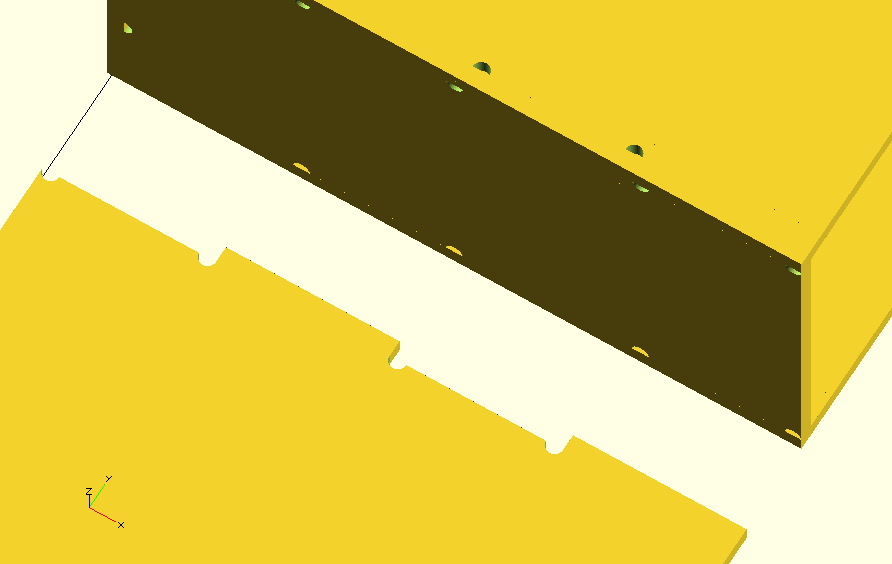## Tabs Held in Place by Screws

Simple through tab, held in place by a screw. Paarameters are direction, x, y, Screw-size) so for example [UP, 50, 100, 3] - for a M3 screw to hold the tab in place.

``````include <../lasercut.scad>;

thickness = 3.1;
x = 50;
y = 100;

\$fn=60;

module base()
{
lasercutoutSquare(thickness=thickness, x=x, y=y,
screw_tabs=[
[UP, x/2, y, 3]
]
);
}

module side()
{
rotate([90,0,0])
lasercutoutSquare(thickness=thickness, x=x, y=y,
screw_tab_holes=[
[MID, x/2-thickness/2, thickness, 3]
]
);
}

translate([0,0,0]) base();
translate([0,y+thickness+20,-thickness]) side();
translate([x+20,0,0]) base();
translate([x+20,y+thickness,-thickness]) side();
``````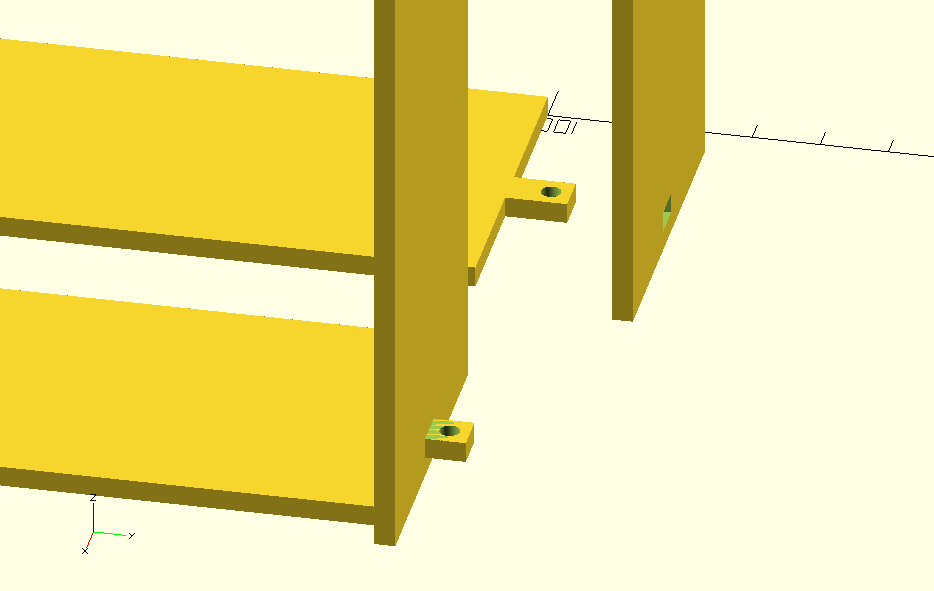## Twist Fit

Example joint, copied with permission from http://msraynsford.blogspot.co.uk/2012/06/panel-joinery-10.html from Martin Raynsford's Blog. This joint is formed by placing one part through the other and rotating it into place.

Usual parameters (direction, x, y, spine=optional). Spine is option and determines the length of the central spine, default is three times thickness.

``````include <../lasercut.scad>;

// From http://msraynsford.blogspot.co.uk/2012/06/panel-joinery-10.html

\$fn=60;
thickness = 3.1;
x = 100;
y = 150;
x_beam = 50;

module beam()
{
lasercutoutSquare(thickness=thickness, x=x_beam, y=y,
twist_connect=[
[RIGHT,x/2,y/2],
[RIGHT,x/2,y/4,x_beam/2]
]
);
}

module crossBeam()
{
rotate([90,0,0])
lasercutoutSquare(thickness=thickness, x=x, y=y,
twist_holes=[
[RIGHT, x/2, y*3/4, x_beam, x_beam/2],
[UP, x/2, y/4, x_beam]
]
);
}

rotate([-90,0,0])  crossBeam();
translate([x+thickness,0,0]) beam();

translate([0,y+75,0]) crossBeam();
translate([(x-x_beam)/2,y/2+75-thickness,y*1/4-thickness/2]) rotate([0,0,0]) beam();
translate([x/2-thickness/2,y*3/4+75-thickness,y*3/4+x_beam/2]) rotate([0,90,0]) beam();

``````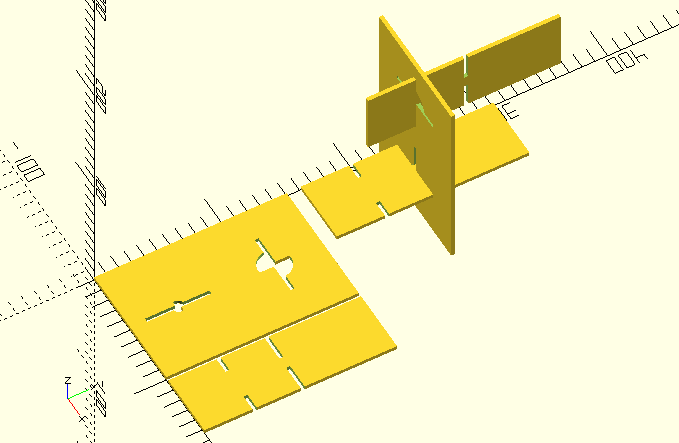## Clip

Loosley based upon http://www.deferredprocrastination.co.uk/blog/2013/so-whats-a-practical-laser-cut-clip-size/ the clip and mathcing hole. Should be good for acrylic, mdf but maybe not plywood.

This is quite a long connnector (thickness * 11) to allow the material to bend and clip in place.

``````include <../lasercut.scad>;

\$fn=60;
thickness = 3.1;
x = 50;
y = 75;
x_beam = 20;

module beam()
{
lasercutoutSquare(thickness=thickness, x=x_beam, y=y,
clips=[
[UP,x_beam/2,y]
]
);
}

module crossBeam()
{
rotate([90,0,0])
lasercutoutSquare(thickness=thickness, x=x, y=y,
clip_holes=[
[DOWN, x/2, y/4, x_beam]
]
);
}

rotate([-90,0,0])  crossBeam();
translate([x+thickness,0,0]) beam();

translate([0,y*3/2+50,0]) crossBeam();
translate([(x-x_beam)/2,y/2+50-thickness,y/4]) rotate([0,0,0]) beam();
``````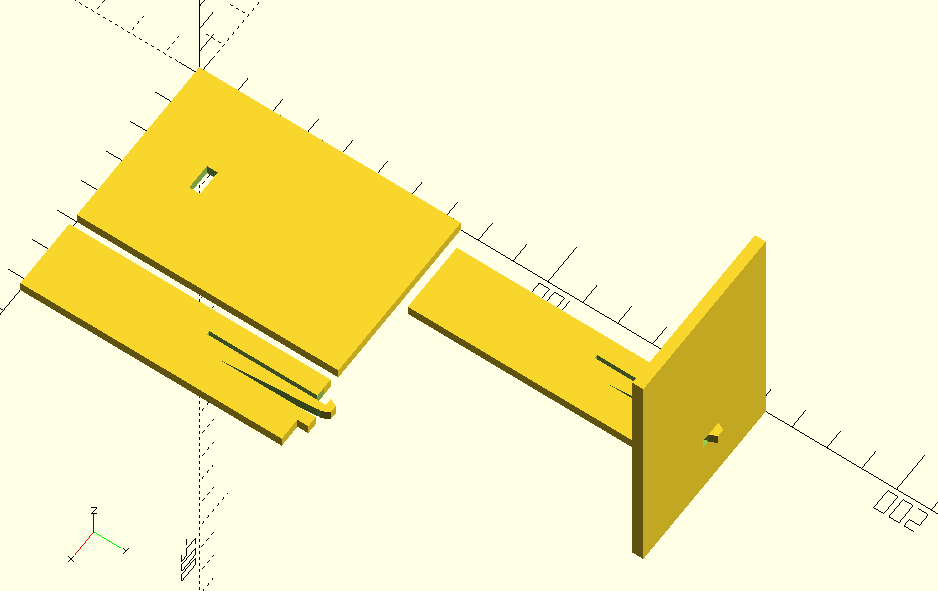## Add or remove circles, make a slit or cut-out a rectangle

Circles are [radius, x, y] Slits are [direction,x,y,length of slit] Cutouts are [x, y, width of cutout, height of cutout]

``````include <lasercut.scad>;

thickness = 3.1;
x = 50;
y = 100;
r = thickness*2;
lasercutoutSquare(thickness=thickness, x=x, y=y,
finger_joints=[
[UP, 1, 4],
[DOWN, 1, 4]
],
[r, x+thickness, y/5],
],
circles_remove = [
[r, x/2, y/2],
[1.5, x/2, y*2/3], // Screw-hole
],
slits = [
[RIGHT,x,y/2,10]
],
cutouts = [
[x/6, y/6, thickness*5, thickness*2]
]
);

``````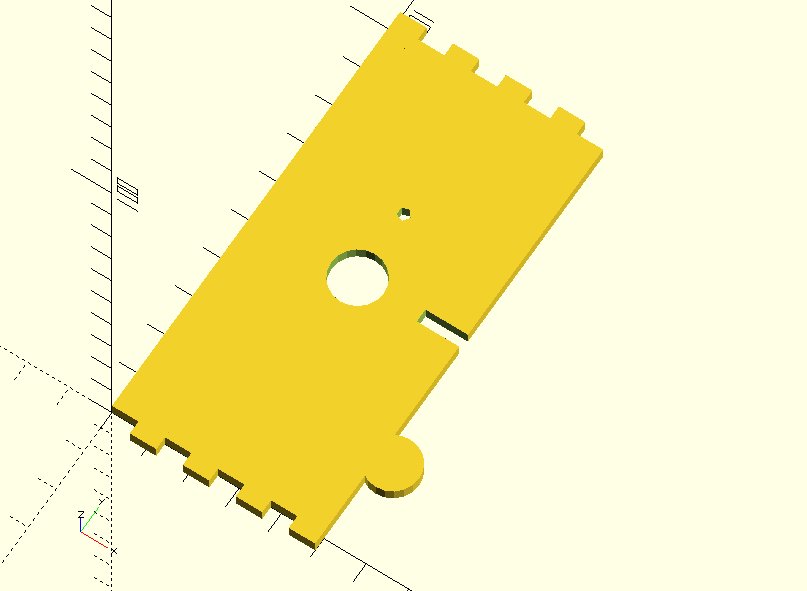## Complex Example

Putting these all together - gives a better example - https://github.com/bmsleight/lasercut/blob/master/examples.scad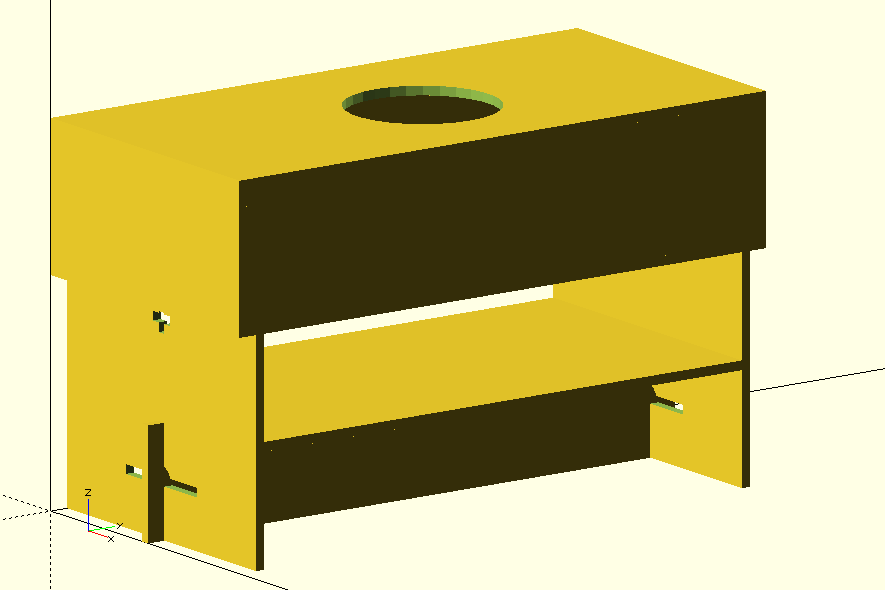## Complex Examples - Box is an array

The box is made of 4,5 or 6 sides. Each of the sides can have any of slips, tab or cutout circles.

## Automatically Generate Files Ready for Laser-Cutter

These models are all very nice, but we need have a nice file, normally dxf to use in a laser-cutter. So we can do this automatically.

``````/bin/bash ./convert-2d.sh examples.scad
``````

This generates three files;

This is a openscad file showing all the shapes along the y-axis.

To adjust the positions of the cut-out, so that are not all along the y-axis the parameter flat_adjust can be used, either in the main files or tweaked in the 2d_ file. For example flat_adjust = [110, -350]), would put the next peice at 110 in x direction and -250 in y-direction. With just using flat_adjust four times, we get a more practical example.

Which give an arrangement and can be exported to a new dxf file.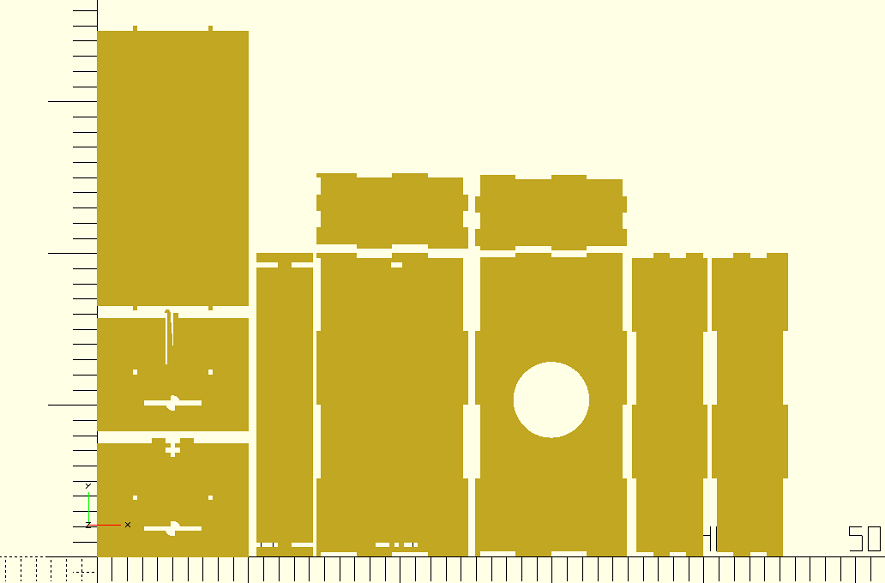## No Laser-Cutter, use Vinyl Cutter

``````include <lasercut.scad>;

rotate([0,270,0]) lasercutoutVinylBox(x=80, y=60, z=50, thickness=0.2);
``````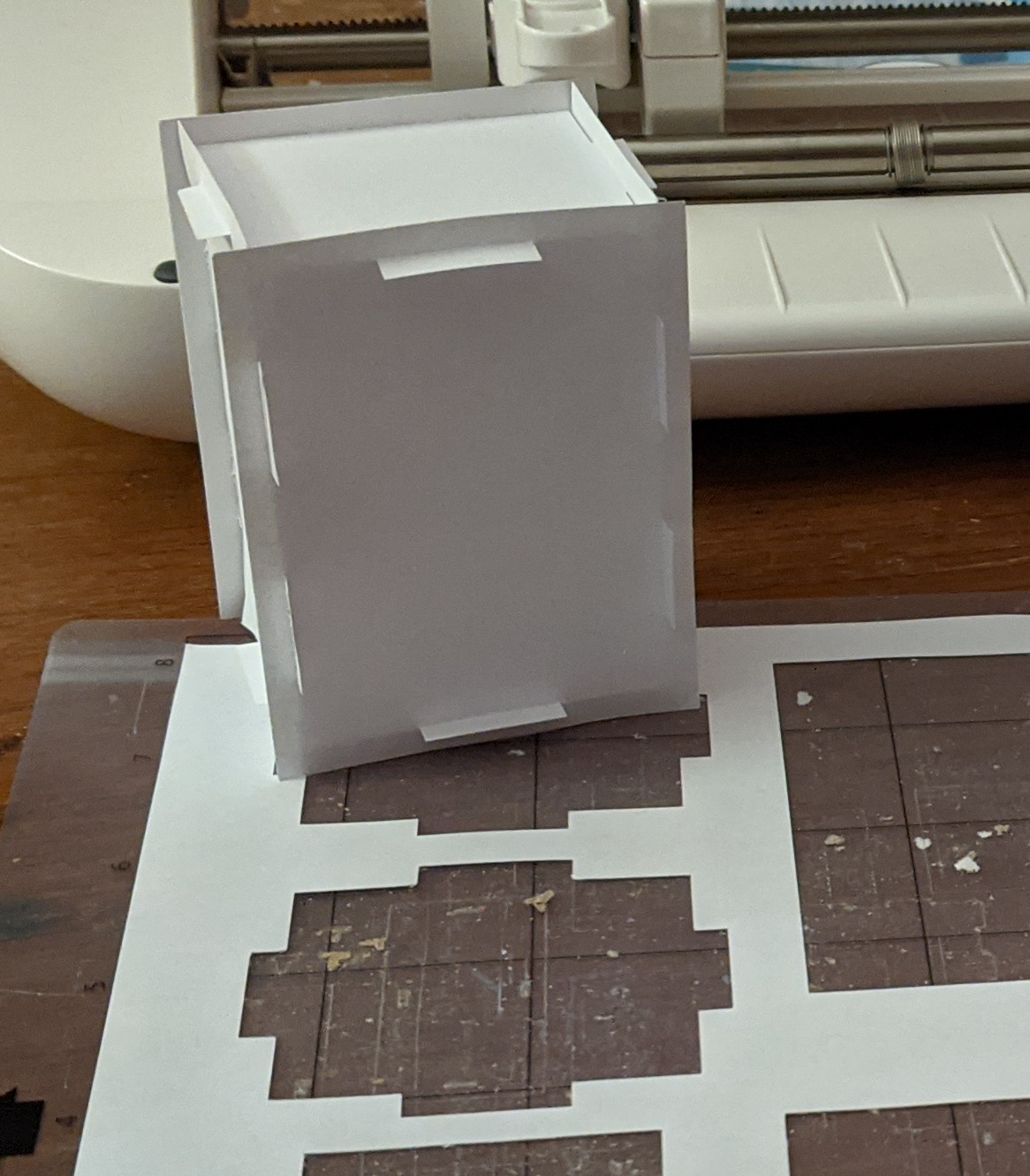Module for openscad, allowing 3d models to be created from 2d lasercut parts